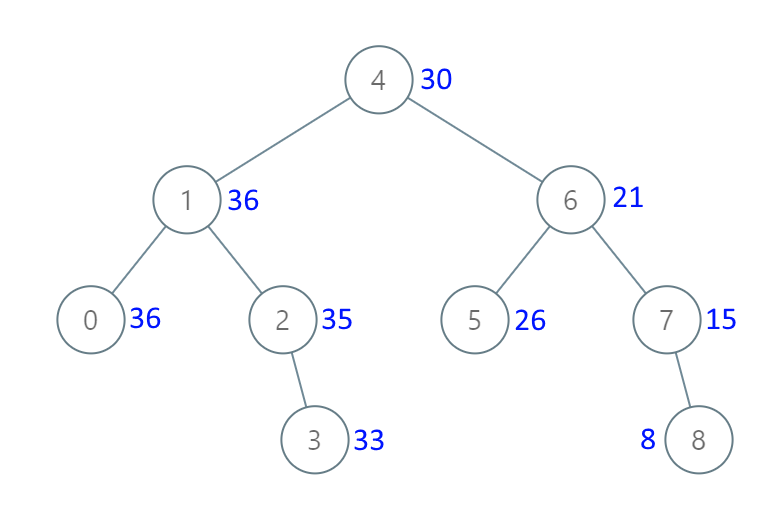# Leetcode 538 - Convert BST to greater tree

Note:

• Wasted too much time on a wrong solution even if I knew that I should do reverse inorder traversal. Need to pratice more binary tree questions using recursion.
• We just need a global variable acc to store sums. That’s it.

Given the root of a Binary Search Tree (BST), convert it to a Greater Tree such that every key of the original BST is changed to the original key plus the sum of all keys greater than the original key in BST.

As a reminder, a binary search tree is a tree that satisfies these constraints:

• The left subtree of a node contains only nodes with keys less than the node’s key.
• The right subtree of a node contains only nodes with keys greater than the node’s key.
• Both the left and right subtrees must also be binary search trees.

Example